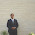VHDL coding tips and tricks: Recursive functions in VHDL

## Monday, April 19, 2010

### Recursive functions in VHDL

In this article I will write about recursive functions in VHDL.Recursion is a method of defining functions in which the function being defined calls itself. The idea is to execute a task in a loop, in a self similar way.
When comes to VHDL, you cannot blindly write a recursive code in your design.Recursive logic has the disadvantage of eating up lot of resources in FPGA. I have explained these points with the help of an example.

Let us see what is the objective of the code,I have written.Here I am doing repetitive XOR operation on a 16 bit signal,until the result obtained is 2 bit. For example if the signal is "1111001100010101" ,then I am doing the following steps:
MSB 8 bits of signal : 11110011
LSB 8 bits of signal  : 00010101
XOR operation        : 11100110   ( call this signal as 'x1')

MSB 4 bits of x1 : 1110
LSB 4 bits of x1  : 0110
XOR operation   : 1000   ( call this signal as 'x2')

MSB 2 bits of x2 : 10
LSB 2 bits of x2  : 00
XOR operation   : 10   ( this should be the result obtained when the code is simulated)

Now normally if you want to do such a program you have to define a lot of temporary signals for x1,x2 etc.This makes the code more complicated and difficult to understand.
But to make the code short and simple ,you can write a recursive function like shown below:

library IEEE;
use IEEE.STD_LOGIC_1164.ALL;

entity recursion is
port ( num : in std_logic_vector(15 downto 0);
exor_out : out std_logic_vector(1 downto 0)
);
end recursion;

architecture Behavioral of recursion is

function exor( num : std_logic_vector ) return std_logic_vector is
variable numf : std_logic_vector(num'length-1 downto 0):=(others => '0');
variable exorf : std_logic_vector((num'length/2)-1 downto 0):=(others => '0');

begin
numf := num;
if(num'length = 4) then
exorf := numf(1 downto 0) xor numf(3 downto 2);
else
exorf := exor(numf(num'length-1 downto num'length/2)) xor exor(numf((num'length/2)-1 downto 0));
end if;
return exorf;
end exor;

begin
exor_out <= exor(num);
end Behavioral;

Now let us analyse the code.

1)function exor( num : std_logic_vector ) return std_logic_vector is :
The function is defined in a general sense.This means the function "exor" takes a std_logic_vector" of any length as input and outputs another std_logic_vector.

2)variable numf : std_logic_vector(num'length-1 downto 0):=(others => '0');
This is the signal on which XOR operation has to be performed.The num'length attribute is used to get the size of the input argument.This value is initialized to zero.

3)variable exorf : std_logic_vector((num'length/2)-1 downto 0):=(others => '0');
This is the result of XOR operation.The result always has half the size of the input.

4) if(num'length = 4) then
exorf := numf(1 downto 0) xor numf(3 downto 2);
else
exorf := exor(numf(num'length-1 downto num'length/2)) xor exor(numf((num'length/2)-1 downto 0));
end if;
On the 4th line, I am calling the function exor in a recursive way.Each time the function is called only half of the signal gets passed to the function.The recursive call is continued until the size of the signal becomes 4.Note that how, exor function calls itself , each time passing only  a part of the input applied to it until it reaches a critically small size(which is 4 here).

Now let us see how this code is synthesised.The Technology schematic view of the design is shown below:
By analyzing the figure you can see that there are 4 LUT's used to implement the logic-Two 4 input  LUT's and two 5 input LUT's.the connection can be understood from the below block diagram:
From the figure you can see that for implementing the logic relativly more resources are used.This is the disadvantage of recursive functions in VHDL. In C and other high level languages recursion is implemented using stack and, there the main issue is stack overflow.But in a HDL like VHDL, the resources may get heavily used for even simple codes.The synthesis tool implements the logic by replicating the function in separate hardware components.This means that if a function calls 10 times itself, then the resources will be used nearly 10 times than that , when an individual block is implemented.
The same thing if we implement with the help of a clock,then you need to use only two XOR gates.But in that case the block will take 8 clock cycles to compute the output.But the recursive function defined here uses more logic resources to compute the output in less time.

Note :- Use recursive functions when you have enough logic gates in your FPGA, and speed is your main concern.

#### 6 comments:

1.I do not agree that recursive function programming uses more resources that the more used for - loop or so. I have several simple examples where the recursive method generates the same logic usage, but where the RTL diagram is much smaller and a lot easier to understand.

2.@Josy : I didnt say that recursive functions uses more logic gates than loops.They uses the same amount of logic.But if you keep the logic clock controlled then the logic gate usage will be lesser.
You can post some examples if you have.

3.@ josy - please post few examples about what you said

4.¿Why don't you use num directly in place of numf?

5.@William : you can use num.I just used numf. There is no particular reason behind it.

6.can any body tell me how to generate gray code directly without using binary counter or exor gates
since we say gray code is having lesser transitions but if we use conversion intermediately we are using binary counter to generate the gray. if we use direct gray increment-er that logic toggle increases,
then how can we say we have low power dissipation in gray counter